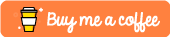#Memoization For Beginners

In this tutorial we are going to look at a concept in computer science called `memoization`. This is a really cool concept that allows us to optimize the runtime performance of some of our recursive algorithms by effectively caching the results of previous computations so that they don’t have to be continuously re-computed.

# The Fibonacci Example

Calculating Fibonacci in a recursive manner is quite possibly the best example I’ve come across when it comes to showing the power of `memoization`.

Imagine we had a function that computed the fibonacci number `n` like so:

``````# Basic fibonacci function
# 1 + 1 + 2 + 3 + 5 + 8 + 13
def fib(n):
if n <= 0:
return 0
if n == 1:
return 1
return fib(n-1) + fib(n-2)``````

When we then tried to run our `fib(7)` function it would then compute the following answer.

``````>>> import fibonacci
>>> fibonacci.fib(7)
13``````

Now the runtime complexity of this relatively simple function above would be `O(2^n)` which is incredibly inefficient as we start to compute larger and larger fibonacci numbers.

# The Memoization Optimization

Through the use of `memoization` we could effectively store the results of previous computations. In order to store our results we will use a `dict` in Python.

``````def fib_with_memo(n, memo):
if n <= 0:
return 0
if n == 1:
return 1
if n not in memo:
print("Memo Computed")
memo[n] = fib_with_memo(n-1, memo) + fib_with_memo(n-2, memo)

return memo[n]

memo = {}

print(fib_with_memo(9, memo))
print(memo)``````

When you run this you should get the following output. As you can see from the number of times `Memo Computed` is printed out, we have been able to effectively optimize the number of times we have to recursively call `fib()`.

`````` \$ python3.6 fibonacci.py
Memo Computed
Memo Computed
Memo Computed
Memo Computed
Memo Computed
Memo Computed
Memo Computed
Memo Computed
34
{2: 1, 3: 2, 4: 3, 5: 5, 6: 8, 7: 13, 8: 21, 9: 34}``````

We have been able to modify our program and change is runtime performance from `O(2^N)` to `O(N)` which is a huge saving.

# Entire Program

The entire Python file can be found below.

``````import time

def fib(n):
if n <= 0:
return 0
if n == 1:
return 1
return fib(n-1) + fib(n-2)

def fib_with_memo(n, memo):
if n <= 0:
return 0
if n == 1:
return 1
if n not in memo:
memo[n] = fib_with_memo(n-1, memo) + fib_with_memo(n-2, memo)

return memo[n]

memo = {}

start_time = time.time()
print(fib(35))
end_time = time.time()
print("Total Time: {}".format(end_time - start_time))

start_time = time.time()
print(fib_with_memo(35, memo))
end_time = time.time()
print("Total Time: {}".format(end_time - start_time))

print(memo)``````

The outputs of this are:

`````` \$ python3.6 fibonacci.py
9227465
Total Time: 7.323758840560913
9227465
Total Time: 0.00010204315185546875
{2: 1, 3: 2, 4: 3, 5: 5, 6: 8, 7: 13, 8: 21, 9: 34, 10: 55, 11: 89, 12: 144, 13: 233, 14: 377, 15: 610, 16: 987, 17: 1597, 18: 2584, 19: 4181, 20: 6765, 21: 10946, 22: 17711, 23: 28657, 24: 46368, 25: 75025,26: 121393, 27: 196418, 28: 317811, 29: 514229, 30: 832040, 31: 1346269, 32: 2178309, 33: 3524578, 34: 5702887, 35: 9227465}``````

As you can see the `memoized` version of the fibonacci function returns in a fraction of the time.

# Conclusion

If you found this tutorial useful or need further explanation then please feel free to let me know in the comments section below!